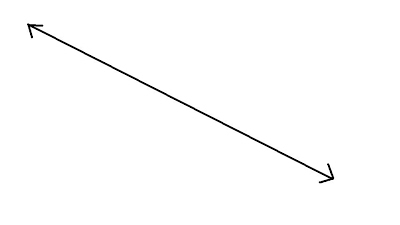100

12 x 3=

36

100What is this called?

A line

100

1/4 + 2/4=

3/4

100

20 x 20=

400

100

Obtuse

What is an angle that is over 90 degrees?

200

8 x 8=

64

200

A right angle is at what degrees?

90 degrees

200

Change to an improper fraction.

2 1/8

17/8

200

250 x 2 =

500

200

An example of this kind of fraction is

8/6

What is an improper fraction?

300

11 X 7=

77

300
What is the angle called that is less than 90 degree?

Acute

300

Change to a mixed number.

26/3

8 2/3

300

120 + 120 + 20 + 20 + 1=

281

300

Factors

What are the numbers you multiple together?

400

25 x 4 =

100

400

A square has one side that is 32mm long.  What is the perimeter of the square?

128 mm

400

Stella ate 4/2 oranges before she took her medicine and ate another 3/2 oranges afterward. How many oranges did she eat?

3 1/2 oranges

400

4 times 600

2,400

400

Product

What is the answer to a multiplication problem?

600

1,300 x 3 =

1,900

600

A square has one side that is 32mm long.  What is the area of the square?

1,024 square mm

600

Change to an improper fraction.

4 2/7

30/7

600

800 x 20

16,000

600
Part of a whole
What is the definition of a fraction?
Click to zoom